# The Möbius function

 The Möbius function Dilafruz Sh.Usmonova May 29, 2020 Abstract The German mathematician August Ferdinand Möbius introduced in 1832 the classical Möbius function μ(n) , which an important multiplicative function in number theory and combinatorics. In this paper we study Möbius function, Möbius theorem and some properties of this function. Keywords and Phrases : Multiplicative function ,arithmetic function, distinct primes 1 Introduction We define an arithmetical function µ Let us recall a definition of arithmetic function; Definition 1. An arithmetic function is a function defined on the positive integers which takes values in the real or complex numbers.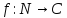Definition 2. An arithmetic function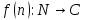is multiplicative if for any relatively prime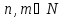: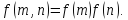Problem If length is equal to , how many words from X with different cycles from x? 2.The Möbius function The Möbius function is an arithmetic function of a natural number argument n with µ(1)=1,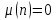if  is divisible by the square of a prime number, otherwise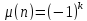,where k  is the number of prime factors of n. Definition. The Möbius function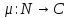is defined by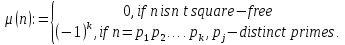This definition and the following expression are equally valid: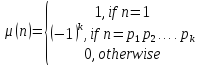Examples.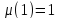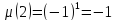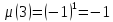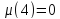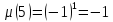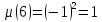Download 25.27 Kb.Do'stlaringiz bilan baham: